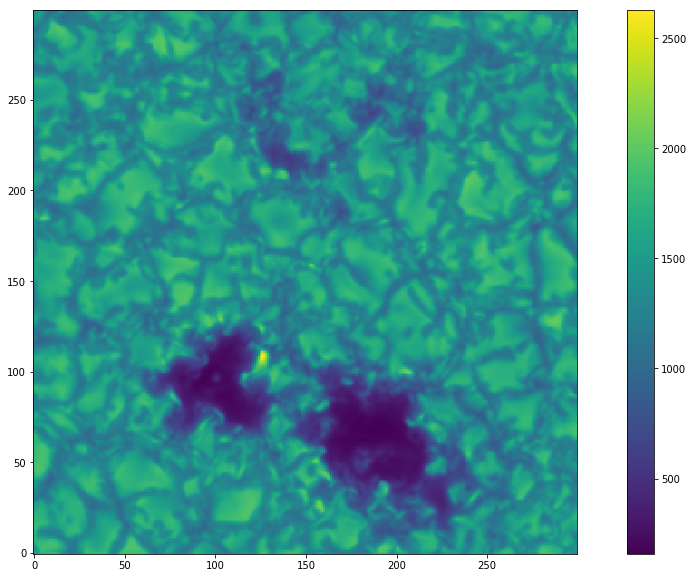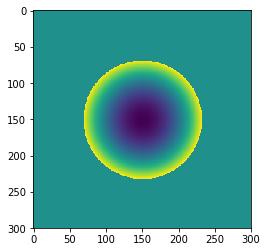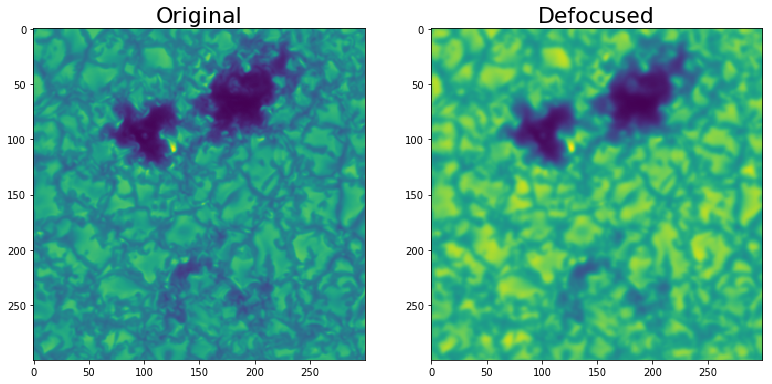# How to use Zernike coefficients to introduce some optical aberrations

## Image Aberration Operations¶

### In this notebook I will illustrate the effect of different optical aberrations on an example image.¶

In :
```import WF_psf_mtf
from WF_psf_mtf import *
import pyfits
import numpy as np
import matplotlib.pyplot as plt
```
In :
```p = '/home/fatima/Desktop/solar_orbiter_project/codes/targets/MTF/'
data = pyfits.getdata(p+'Masi_hrt.fits')
data = data[300:600,0:300]
```
In :
```plt.figure(figsize=(18,10))
plt.imshow(data,origin='lower')
plt.colorbar()
```
Out:
`<matplotlib.colorbar.Colorbar at 0x7f70840e4710>`### We will be working on a sub region of the whole image for computational purposes.¶

#### Declaring the telescope parameters again¶

In :
```D = 140 #diameter of the aperture
lam = 617.3*10**(-6) #wavelength of observation
pix = 0.5 #plate scale
f = 4125.3            #effective focal length
size = data.shape #size of detector in pixels
```

## Defocus¶

#### I will show here how to introduce a defocus to the image using the wavefront aberration analysis described in wavefront to PSF to MTF. The defocus term is the fourth term in the Zernike expansion scheme. Usually the defocus is described in terms of optical path difference in terms of wavelength. So we will define a function that converts an OPD to a Zernike coefficient for¶

In :
```coefficients = np.zeros(8)
coefficients = 0
coefficients = 0
coefficients = 0
coefficients = 0.9
coefficients = 0
coefficients = 0
coefficients = 0
coefficients = 0
```
In :
```rpupil = pupil_size(D,lam,pix,size)
sim_phase = center(coefficients,size,rpupil)
```
In :
```plt.imshow(sim_phase)
```
Out:
`<matplotlib.image.AxesImage at 0x7f7084327a10>`### Let's construct now the corresponding PSF and OTF¶

In :
```pupil_com = complex_pupil(sim_phase,Mask)
psf = PSF(pupil_com)
otf = OTF(psf)
mtf = MTF(otf)
```

### Now we should convolved the simulation with the derived OTF. We multiply the FT of the image with the OTF, then use the inverse FT to derived the blurred image.¶

In :
```fft_image = np.fft.fft2(data)
temp = fft_image*otf
defoc = np.fft.ifft2(temp).real
```
In :
```fig = plt.figure(figsize=(15,10))
im=ax.imshow(data)
im2=ax2.imshow(defoc)
ax.set_title('Original',fontsize=22)
ax2.set_title('Defocused',fontsize=22)
#cbar_ax = fig.add_axes([0.85, 0.15, 0.05, 0.7])
#fig.colorbar(im, cax=cbar_ax)
```
Out:
`<matplotlib.text.Text at 0x7f7083ae4ad0>`## Image Misalignment¶

#### Let's show here how to introduce image shifts to the original. This shift can be done by including the tip/tilt parameters (first 3 parameters), the OTF of which will result in geometrical translation in both x and y directions.¶

In :
```coefficients = np.zeros(8)
coefficients = 0.5
coefficients = 2
coefficients = 21
coefficients = 0
coefficients = 0
coefficients = 0
coefficients = 0
coefficients = 0
```
In :
```sim_phase = center(coefficients,size,rpupil)
psf = PSF(pupil_com)
otf = OTF(psf)
mtf = MTF(otf)
```
In :
```fft_image = np.fft.fft2(data)
temp = fft_image*otf
shifted = np.fft.ifft2(temp).real
```
In :
```fig = plt.figure(figsize=(15,10))
`<matplotlib.text.Text at 0x7f70818c6290>`